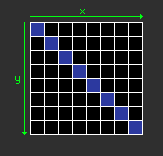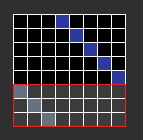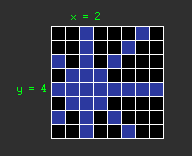# Masquerade ball with eight queens

2011-04-16

A while ago, I had some fun writing a solution for the classic eight queens puzzle. But rather than focusing on the recursive issue which is finally just a dozen of SLOC, I played with the board representation in memory. The recursive algorithm won't be much detailed here, it's not the aim of this post.

## Plate declaration

We need a 8x8 array to represent the plate, so instinctively, we start with the following declaration:

``````int t;
``````

Then, we realized a two-dimensional array is not specially useful since we'll brute-force with an index from 0 to 63:

``````int t;
``````

An `int` is a bit overkill for a `boolean` value, isn't it?

``````uint8_t t;
``````

Hey, maybe could just use 8 bits per line:

``````uint8_t t;
``````

Right, so we have 8x8 bits, then let's use a simpler representation:

``````uint64_t t;
``````

Here we go, much better. We can now start.

## Ascii art

Being able to visually represent our board is the first thing to do. Here is an example:

``````static void disp_board(uint64_t t)
{

for (int i = 0; i < 64; i++, mask <<= 1)
printf("%c%c", t & mask ? '@' : '.', (i + 1) % 8 == 0 ? '\n' : ' ');
printf("\n");
}
``````

This function displays:

``````@ . . . . . . .
. . . . @ . . .
. . . . . . . @
. . . . . @ . .
. . @ . . . . .
. . . . . . @ .
. @ . . . . . .
. . . @ . . . .
``````

...for `t = 577094088726155265`, or in binary:

``````00001000 00000010 01000000 00000100 00100000 10000000 00010000 00000001
``````

You'll notice I choose the LSB for the bit corresponding to the position `(0, 0)` on the board (`1` means a queen, `0` means no queen).## Recursion

About the recursion, here is the code with the appropriate `main()`:

``````#define sq(i) do { t |=   1ULL << i;  qleft--; } while (0)
#define rq(i) do { t &= ~(1ULL << i); qleft++; } while (0)

static int solve(uint64_t t, int qleft, int idx)
{
int n = 0;

if (idx == 64)
return 0;
if (ok(t, idx)) {
sq(idx);
if (qleft == 0) {
disp_board(t);
n = 1;
} else {
n = solve(t, qleft, (idx / 8 + 1) * 8);
}
rq(idx);
}
return n + solve(t, qleft, idx + 1);
}

int main()
{
printf("%d\n", solve(0, 8, 0));
return 0;
}
``````

A few notes on this code:

• The macros `sq` and `rq` (respectively `set queen` and `remove queen`) set and unset the queen's bit with index `idx`, and increment/decrement the number of left queens to place.
• The `ok()` function we will study soon let you know if it's possible to put a queen at index `idx` or not.
• The `main()` function runs the recursion with an empty board (`t=0`), eight queens left to place (`qleft=8`) starting at position `idx=0`, or `(0,0)`.
• When all the queens are set, we display the plate, and we continue.
• `solve()` returns the number of solutions when we found and displayed them all.

The fun can now begin with the writing of the last function:

``````static int ok(uint64_t t, int idx);
``````

To simplify a few operations, we fetch the `x` and `y` coordinates:

``````static int ok(uint64_t t, int idx)
{
int y = idx / 8;
int x = idx - y * 8;

// ...
}
``````

And the function's return will look like something like this:

``````return !(t & (MASK_LINE | MASK_COLUMN | MASK_DIAGONAL1 | MASK_DIAGONAL2));
``````

All the masks will form a bit field in which no queen should be. A simple binary `AND` with the board will allow to say if it's the case or not.

Let's start by determining `MASK_LINE` for a given `idx`. A mask for the line could be the following:

``````#define MLI 0x00000000000000ffULL /* 00000000 00000000 00000000 00000000 00000000 00000000 00000000 11111111 */
``````

This mask allow to scratch the first line of the board, so we need to shift it to the given queen line:

``````#define MASK_LINE(y) (MLI << (y) * 8)
``````And in the same way for the columns starting with the left one:

``````#define MCL 0x0101010101010101ULL /* 00000001 00000001 00000001 00000001 00000001 00000001 00000001 00000001 */
``````

Since we have the two masks for lines and columns, we now need to do the diagonals. Let's take the first case:If we take the case where `x > y`, we have to shift to the left (the right on the board) eight time the difference:However, the diagonal gets extended (red frame) while it shouldn't, so we need to filter that part. For this diagonal and `x > y`, we can use the following mask:

``````d = x - y
(MD1 & ~0ULL >> 8 * d) << d
^ ^^^^^    ^^^^^  ^^^^
|  |        |      |
|  |        |      `-- 4. And we shift the diagonal.
|  |        |
|  |        `-- 2. ...we shift them in order to create zeroes in the lowest part of the board.
|  |
|  `-- 1. All bits to 1...
|
`-- 3. We only keep higher part of the board.
``````

With `MD1` (mask for the middle diagonal):

``````#define MD1 0x8040201008040201ULL /* 10000000 01000000 00100000 00010000 00001000 00000100 00000010 00000001 */
``````

To handle the case where `x < y`, we just have to shift in the other direction, and toggle the difference sign:

``````d = x - y
(d > 0 ? (MD1 & ~0ULL >> 8 * d) << d : (MD1 & ~0ULL << 8 * -d) >> -d)
^^^^^^^^^^^^^^^^^^^^^^^^^^^   ^^^^^^^^^^^^^^^^^^^^^^^^^^^^^^
Shift to the right on the     Shift to the left on the board.
board.
``````

The case for the second diagonal is almost the same; the difference is not obtained with `x - y` but `x + y - 7`, and the mask change:

``````d1 = x - y
d2 = x + y - 7

First  diagonal: (d1 > 0 ? (MD1 & ~0ULL >> 8 * d1) << d1 : (MD1 & ~0ULL << 8 * -d1) >> -d1)
Second diagonal: (d2 > 0 ? (MD2 & ~0ULL << 8 * d2) << d2 : (MD2 & ~0ULL >> 8 * -d2) >> -d2)
``````

With the following `MD2`:

``````#define MD2 0x0102040810204080ULL /* 00000001 00000010 00000100 00001000 00010000 00100000 01000000 10000000 */
``````

If we now merge our four masks (line, column, diagonal 1 and diagonal 2), we get this code:

``````#define MLI 0x00000000000000ffULL /* 00000000 00000000 00000000 00000000 00000000 00000000 00000000 11111111 */
#define MCL 0x0101010101010101ULL /* 00000001 00000001 00000001 00000001 00000001 00000001 00000001 00000001 */
#define MD1 0x8040201008040201ULL /* 10000000 01000000 00100000 00010000 00001000 00000100 00000010 00000001 */
#define MD2 0x0102040810204080ULL /* 00000001 00000010 00000100 00001000 00010000 00100000 01000000 10000000 */

static int ok(uint64_t t, int idx)
{
int y  = idx / 8;
int x  = idx - y * 8;
int d1 = x - y;
int d2 = x + y - 7;
return !(t & ((MLI << y * 8) | (MCL << x)
| (d1 > 0 ? (MD1 & ~0ULL >> 8 * d1) << d1 : (MD1 & ~0ULL << 8 * -d1) >> -d1)
| (d2 > 0 ? (MD2 & ~0ULL << 8 * d2) << d2 : (MD2 & ~0ULL >> 8 * -d2) >> -d2)));
}
``````

The result is a binary `AND` between the memory board, and a mask which looks like for example:## Conclusion

We can do better, with less than 64 bits, but I found this approach entertaining (and I got this last link when I was almost done with my code).

For those interested, here is the complete code:

``````#include <stdio.h>
#include <stdint.h>

static void disp_board(uint64_t t)
{

printf("t = %llx\n", t);
for (int i = 0; i < 64; i++, mask <<= 1)
printf("%c%c", t & mask ? '@' : '.', (i + 1) % 8 == 0 ? '\n' : ' ');
printf("\n");
}

#define MLI 0x00000000000000ffULL /* 00000000 00000000 00000000 00000000 00000000 00000000 00000000 11111111 */
#define MCL 0x0101010101010101ULL /* 00000001 00000001 00000001 00000001 00000001 00000001 00000001 00000001 */
#define MD1 0x8040201008040201ULL /* 10000000 01000000 00100000 00010000 00001000 00000100 00000010 00000001 */
#define MD2 0x0102040810204080ULL /* 00000001 00000010 00000100 00001000 00010000 00100000 01000000 10000000 */

static int ok(uint64_t t, int idx)
{
int y  = idx / 8;
int x  = idx - y * 8;
int d1 = x - y;
int d2 = x + y - 7;
return !(t & ((MLI << y * 8) | (MCL << x)
| (d1 > 0 ? (MD1 & ~0ULL >> 8 * d1) << d1 : (MD1 & ~0ULL << 8 * -d1) >> -d1)
| (d2 > 0 ? (MD2 & ~0ULL << 8 * d2) << d2 : (MD2 & ~0ULL >> 8 * -d2) >> -d2)));
}

#define sq(i) do { t |=   1ULL << i;  qleft--; } while (0)
#define rq(i) do { t &= ~(1ULL << i); qleft++; } while (0)

static int solve(uint64_t t, int qleft, int idx)
{
int n = 0;

if (idx == 64)
return 0;
if (ok(t, idx)) {
sq(idx);
if (qleft == 0) {
disp_board(t);
n = 1;
} else {
n = solve(t, qleft, (idx / 8 + 1) * 8);
}
rq(idx);
}
return n + solve(t, qleft, idx + 1);
}

int main()
{
printf("%d\n", solve(0, 8, 0));
return 0;
}
``````

For updates and more frequent content you can follow me on Mastodon. Feel also free to subscribe to the RSS in order to be notified of new write-ups. It is also usually possible to reach me through other means (check the footer below). Finally, discussions on some of the articles can sometimes be found on HackerNews, Lobste.rs and Reddit.